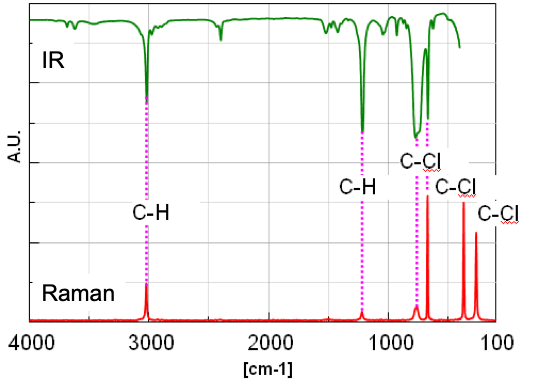# IR/NIR/FT-Raman Measurement using Broadband KBr Beam Splitter (B/S)

November 10, 2022

## Introduction

Heparins Infrared (MIR or NIR) and Raman spectroscopy are widely used for qualitative and quantitative analysis for polymer, food, medicine and semiconductor industries; with the combination of these techniques, it is possible to obtain more information about physical properties of the sample. For example, if MIR and NIR spectroscopy are combined, the following information can be obtained.

• Dissociation energy of molecule (De)
• Absorption index in the IR and NIR region (α)
• Optical constant (n, k)

These values can provide the important perception for prediction of the chemical reaction or design of various devices. Additionally, if IR and Raman spectroscopy are combined, the following information can be obtained.

• Complementary information on molecular vibration
• Information on lattice vibration by measurement in low wavenumber range (specific to Raman spectroscopy)
• Information on molecular vibration when combined with heavy atom

FTIR instrument can measure the IR/Near IR/Far IR spectrum by selecting the proper light source, detector, and B/S, and if the FT-Raman system is used, Raman spectrum  can also be obtained. The measurement range covered by general B/S used in FTIR is from 7800 to 375 cm-1, while the newly developed broadband KBr B/S covers the range from 12000 to 375 cm-1, enabling the measurement of IR/NIR/Raman spectrum without replacing the B/S. This application data shows the perception of the basic physical property of the chloroform by measuring the IR/NIR/Raman spectrum using such broadband KBr B/S.

## Experimental

The IR and NIR spectrum are measured by the system as shown in Figure 1 under the conditions as shown in Table 1.

Table 1 Measuring Conditions

 Measurement Range (cm-1) Light pathlength of liquid cell Light source* Detector B/S 4000~400 0.025 mm Ceramic DLATGS Broadband 6400~4000 1 mm Halogen 12000~6400 5 mm

* Raman Spectrum: Ex wavelength: 1064 nm, Detector: InGaAs

Calculation of Dissociation energy (De) and Absorption index (α) of C-H by using IR and NIR spectrum

According to the general interpretation of the IR spectrum, the harmonic oscillator model is employed in which the atoms constituting the molecule are combined by spring (Dotted line by simple quadratic function in Figure 2), however, it is impossible to explain the overtone and combination tone in NIR region by this harmonic oscillator model. Therefore, in order to interpret the NIR spectrum, the Morse function in which the anharmonic

oscillation is taken into consideration (Continuous line in Figure 2)1). In the Morse function, the energy value is becoming close to Dissociation energy (De) asymptotically when interatomic distance increases. In this case, the De value of C-H is calculated by using absorption wavenumber of stretching vibration v1, 2v1, 3v1) of chloroform and Morse function. The Absorption index (α)** is also calculated by absorbance (Abs) at each wavenumber of. The IR/NIR spectrum of chloroform and attribution of each peak are shown in Figure 3.

The dissociation energy (De) of C-H in chloroform is calculated to be 460 +/- 7 kJ/mol from equation 2, equation 4, and value of absorption wavenumber of stretching vibration of C-H (v1, 2v1, 3v1)2).

The absorption index α of n1, 2v1, 3v1 is calculated from measured spectra to be 219, 15.5, and 0.56 respectively as shown in Table 2. From those results, for general organic substances, it is estimated that the sample with high concentration can be quantitated, such as about several 10 times if 2v1 is used and about several 100 times if 3v1 is used. Therefore, in NIR range, it is possible to measure the samples in non-destructive method, without dilution which is required for IR measurement.

Table 2 Absorbance index and assignment of Chloroform

Wavenumber (cm-1) Assignment Abs α**
3020v1 (C-H stretching vibration)0.55219
1215v4 (C-H bending vibration) 2.02806
5375v1+2v40.191.88
59092v11.5515.5
70892v1+v40.651.30
86743v10.280.56

** α: Absorbance if the light path is 1 cm for this experimentFig. 4 IR and FT-Raman spectrum of Chloroform(Vertical scale: A.U. due to showing %T and Int., Horizontal scale: [cm-1] for wavenumber and Raman shift.)

Figure 4 shows the IR spectrum (Vertical axis: %T) and FT-Raman spectrum (Vertical axis: Int.). The peak intensity ratio in IR spectrum between v1 and v4 of C-H is about 3.68 from Table 2. Regarding this, since the intensity ratio in Raman spectrum is about 1/4, it is confirmed that the peak intensities of IR and Raman spectrum are complementary. In general, when the change of the dipole moment in the molecular vibration is larger (asymmetric vibration mode), the intensity of peak in IR spectrum appears higher, while the stronger peak in Raman spectrum is detected when the change of the polarizability is larger (symmetric vibration mode). Therefore, the change of the dipole moment for n1 of C-H is considered to be larger than the one of v4 (the change of polarizability is smaller). The vibration of molecule combined with the heavy atom like C-Cl can be considered to be detected in the range less than 400 cm-1 from Raman spectrum. It is important to select IR or FT-Raman depending on the vibration mode even if the structure of molecule is simple like Chloroform.

## Keywords

FT-Raman, Extended range, Chloroform, FTIR, NIR

## Results

This application data shows one of the topics in vibrational spectroscopy taking the Chloroform for example, using broadband KBr B/S. Besides, it can be considered that as well as the general qualitative and quantitative analysis, the broadband KBr B/S can be applied to the 2D correlated spectroscopy based on chemical reaction analysis by time-course measurement using 3 measurement methods. By using broadband KBr B/S which does not require replacement, the measurement mode can be switched without breaking vacuum or interrupting N2 purge. This broadband B/S will expand the potential to wider application fields.

## References

Kenneth W. Busch, et,al, Appl. Spectrosc. 54, 1321 (2000)

Chemical Handbook, the revised third edition